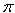SEARCH HOMEMath Central Quandaries & QueriesQuestion from ashley, a student: hello, I've been stumped for hours on this problem and can't quite figure it out. The question is: A tepee is a cone-shaped shelter with no bottom. Suppose you have 200 square feet of canvas (shaped however you like) to make a tepee. Use calculus to find the height and radius of such a tepee that encloses the biggest volume. Can you help??Hi Ashley,

We are going to assume the cone is a right circular cone. The volume of a right circular cone is

V = 1/3r2 h

where r is the radius of the base and h is the height. The surface area of the cone is given by

S =r (h2 + r2)1/2

If this formula is unfamiliar to you then go to the Quandaries and Queries page and search in the Quick Search window for the term cone area to see our answers to similar questions concerning the surface area of a cone. Since you know that S = 200 sq ft you have

200 =r (h2 + r2)1/2

Squaring both sides and solving foe h gives

h = [(40000 -2 r4)/2 r2]1/2

Substitution of this value for h into the expression for the volume gives

V = 1/3 r (40000 -2 r4)1/2

Now use the calculus you know to maximize V.

Stephen and PennyMath Central is supported by the University of Regina and The Pacific Institute for the Mathematical Sciences.